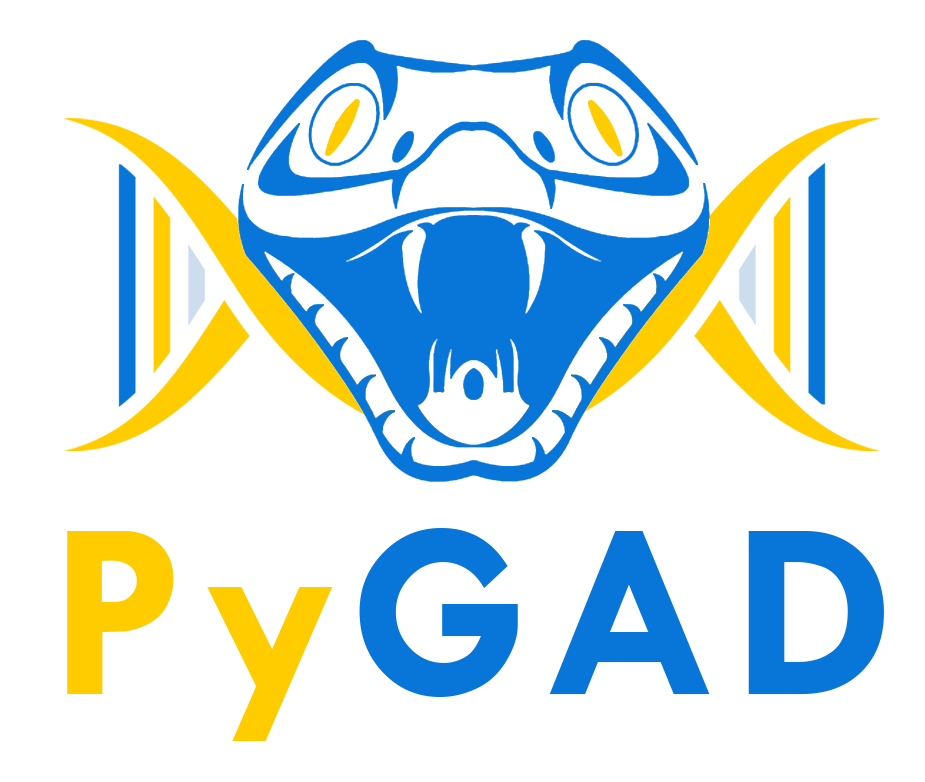# PyGAD - Python Genetic Algorithm!¶

PyGAD is an open-source Python library for building the genetic algorithm and optimizing machine learning algorithms. It works with Keras and PyTorch.

PyGAD supports different types of crossover, mutation, and parent selection operators. PyGAD allows different types of problems to be optimized using the genetic algorithm by customizing the fitness function. It works with both single-objective and multi-objective optimization problems.Logo designed by Asmaa Kabil

Besides building the genetic algorithm, it builds and optimizes machine learning algorithms. Currently, PyGAD supports building and training (using genetic algorithm) artificial neural networks for classification problems.

# Donation & Support¶

You can donate to PyGAD via:

# Installation¶

Install PyGAD with the following command:

```pip3 install pygad
```

PyGAD is developed in Python 3.7.3 and depends on NumPy for creating and manipulating arrays and Matplotlib for creating figures. The exact NumPy version used in developing PyGAD is 1.16.4. For Matplotlib, the version is 3.1.0.

# Quick Start¶

To get started with PyGAD, simply import it.

```import pygad
```

Using PyGAD, a wide range of problems can be optimized. A quick and simple problem to be optimized using the PyGAD is finding the best set of weights that satisfy the following function:

```y = f(w1:w6) = w1x1 + w2x2 + w3x3 + w4x4 + w5x5 + w6x6
where (x1,x2,x3,x4,x5,x6)=(4,-2,3.5,5,-11,-4.7) and y=44
```

The first step is to prepare the inputs and the outputs of this equation.

```function_inputs = [4,-2,3.5,5,-11,-4.7]
desired_output = 44
```

A very important step is to implement the fitness function that will be used for calculating the fitness value for each solution. Here is one.

If the fitness function returns a number, then the problem is single-objective. If a `list`, `tuple`, or `numpy.ndarray` is returned, then it is a multi-objective problem (applicable even if a single element exists).

```def fitness_func(ga_instance, solution, solution_idx):
output = numpy.sum(solution*function_inputs)
fitness = 1.0 / numpy.abs(output - desired_output)
return fitness
```

Next is to prepare the parameters of PyGAD. Here is an example for a set of parameters.

```fitness_function = fitness_func

num_generations = 50
num_parents_mating = 4

sol_per_pop = 8
num_genes = len(function_inputs)

init_range_low = -2
init_range_high = 5

parent_selection_type = "sss"
keep_parents = 1

crossover_type = "single_point"

mutation_type = "random"
mutation_percent_genes = 10
```

After the parameters are prepared, an instance of the pygad.GA class is created.

```ga_instance = pygad.GA(num_generations=num_generations,
num_parents_mating=num_parents_mating,
fitness_func=fitness_function,
sol_per_pop=sol_per_pop,
num_genes=num_genes,
init_range_low=init_range_low,
init_range_high=init_range_high,
parent_selection_type=parent_selection_type,
keep_parents=keep_parents,
crossover_type=crossover_type,
mutation_type=mutation_type,
mutation_percent_genes=mutation_percent_genes)
```

After creating the instance, the `run()` method is called to start the optimization.

```ga_instance.run()
```

After the `run()` method completes, information about the best solution found by PyGAD can be accessed.

```solution, solution_fitness, solution_idx = ga_instance.best_solution()
print("Parameters of the best solution : {solution}".format(solution=solution))
print("Fitness value of the best solution = {solution_fitness}".format(solution_fitness=solution_fitness))

prediction = numpy.sum(numpy.array(function_inputs)*solution)
print("Predicted output based on the best solution : {prediction}".format(prediction=prediction))
```
```Parameters of the best solution : [3.92692328 -0.11554946 2.39873381 3.29579039 -0.74091476 1.05468517]
Fitness value of the best solution = 157.37320042925006
Predicted output based on the best solution : 44.00635432206546
```

1. The main module has the same name as the library `pygad` which is the main interface to build the genetic algorithm.
2. The `nn` module builds artificial neural networks.
3. The `gann` module optimizes neural networks (for classification and regression) using the genetic algorithm.
4. The `cnn` module builds convolutional neural networks.
5. The `gacnn` module optimizes convolutional neural networks using the genetic algorithm.
6. The `kerasga` module to train Keras models using the genetic algorithm.
7. The `torchga` module to train PyTorch models using the genetic algorithm.
8. The `visualize` module to visualize the results.
9. The `utils` module contains the operators (crossover, mutation, and parent selection) and the NSGA-II code.
10. The `helper` module has some helper functions.

The documentation discusses these modules.

# PyGAD Citation - Bibtex Formatted¶

If you used PyGAD, please consider citing its paper with the following details:

```@misc{gad2021pygad,
title={PyGAD: An Intuitive Genetic Algorithm Python Library},